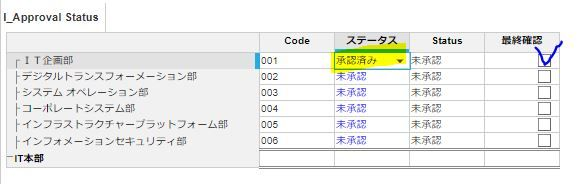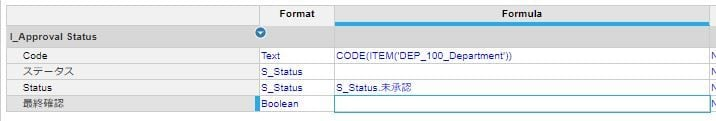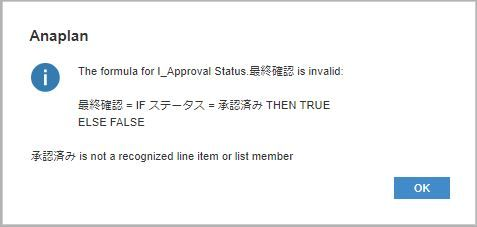# if formula questionHi

I'm trying to make formula but it's not going well

IF ステータス = 承認済み THEN TRUE ELSE FALSE

how can i make this formula?Tagged:

•Hey,

Don't know the language, but understood something

I guess formula should be like

IF name(List formatted line item) = "alert text" THEN TRUE ELSE FALSE or it can be

IF name(List formatted line item) = Line item [Remove name if it's Line item is list format] THEN TRUE ELSE FALSE

You can write formula little better => name(List formatted line item) = "alert text" [No need of use for IF Else condition in this case]

Thanks & Regards,

•Hi @Baek

You need to ensure you're comparing the same data types in your equality.

The line item ステータス is formatted as S_Status, so the right hand side of your equation "承認済み" needs to also be a list formatted line item or value.

If 承認済み is an item in S_Status, then it would need to be rewritten as:

`ステータス = 'S_Status'.承認済み`

As @Sai_Bharadwaj_Venati also said, rather than doing the

`IF ステータス = 'S_Status'.承認済み THEN TRUE ELSE FALSE`

You can just leave it as:

`ステータス = 'S_Status'.承認済み`

Since if this is TRUE, then it will return TRUE, and if it's false it will return FALSE.